# Auto-regression

A regressive dependence of the values of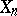of a given random sequenceon the preceding values of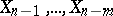. A linear auto-regression scheme of orderis defined by a linear regression equation betweenand,, i.e.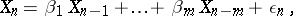(*)
whereare constants and the random variables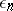are identically distributed with average zero, variance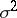and are uncorrelated (sometimes they are assumed to be independent). An auto-regression scheme is a useful stochastic model for the description of certain time series (the concept of a linear auto-regression scheme was first introduced by G. Yule in 1921) in order to analyze time series describing a system which is oscillating under the effect of internal forces and random external shocks. The auto-regression scheme (*) may be regarded as a stochastic process of a special type: an auto-regressive process.